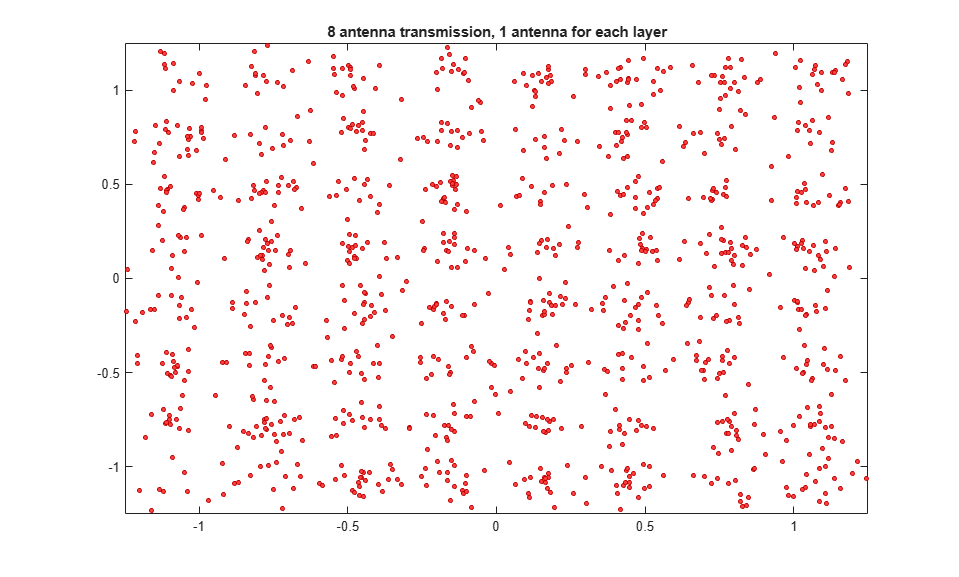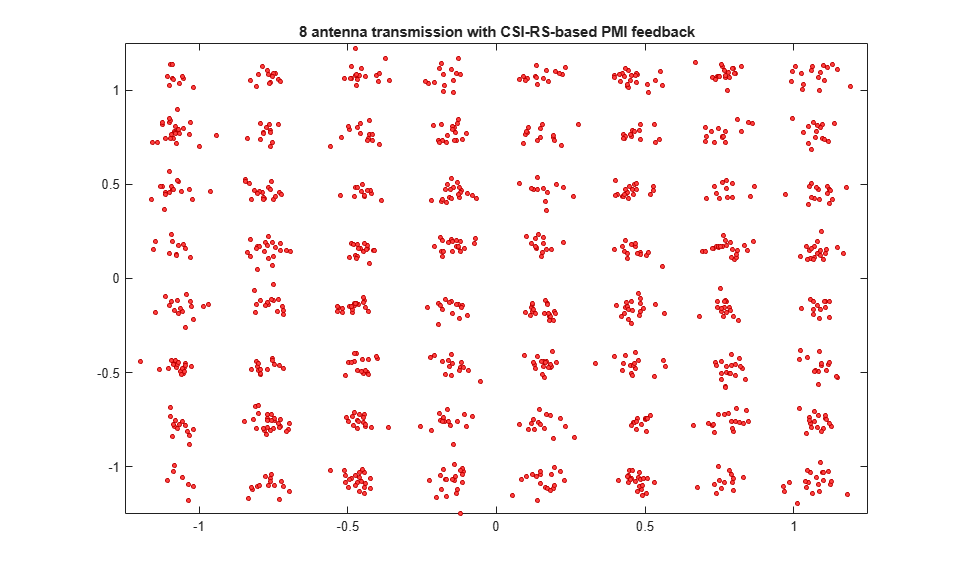# Release 10 PDSCH Enhanced UE-Specific Beamforming

This example demonstrates the Release 10 UE-specific beamforming capability of the LTE Toolbox™ and shows how an appropriate choice of beamforming matrix leads to better performance.

### Introduction

Release 10 allows for transmission of up to 8 layers on antenna ports 7-14 (TS36.213, Section 7.1.5B [ 1 ]). Transmissions in this scheme are made on one or more layers with a reference signal (port) for each layer, and can be beamformed onto any number of transmission antennas using any appropriately-dimensioned beamforming matrix; the choice of the number of transmission antennas and beamforming matrix values are not specified in the standard.

This example shows the Error Vector Magnitude (EVM) improvement achieved when using Precoding Matrix Indicator (PMI) feedback based on channel state information reference signal. A waveform with Physical Downlink Shared Channel (PDSCH) information is created and passed through a noisy fading channel. The received waveform is demodulated, resulting in a received resource grid for each receive antenna. An estimate of the channel is then used to decode the PDSCH, calculate the SNR and singular values of the channel and select an appropriate precoding matrix. The EVM of the received signal is calculated and used to estimate the effective channel SNR. This process is carried out with and without Channel State Information (CSI) and Reference Signal (RS) based PMI feedback to demonstrate the impact on performance.

### Reference Measurement Channel Configuration

Generate Reference Measurement Channel (RMC) configuration structure for RMC R.5; amended to create only one subframe and 5 Resource Blocks (RBs), and use 8 antennas.

Release 10 UE-specific beamforming capability is parameterized within the LTE Toolbox as `TxScheme = 'Port7-14'` in conjunction with the appropriate choice of the number of layers `NLayers`. There are a number of standard defined RMCs using Port7-14 transmission scheme supported by LTE Toolbox. Examples are R.43, R.44, R.45, R.45-1, R.48, R.50 and R.51. See lteRMCDL for details. The beamforming matrix is represented by the PDSCH configuration field `W`; the number of transmission antennas is given by the number of columns of `W`.

```rmc = struct; % RMC config structure rmc.RC ='R.5'; % Base configuration on RMC R.5 rmc.DuplexMode = 'TDD'; % User Time Division Duplex (TDD) rmc.TotSubframes = 1; % Configure a single subframe % Generate the base configuration from RMC R.5 and amend to set the % parameters required for the Port7-14 transmission scheme. Note that if % the standard defined RMCs using Port7-14 transmission scheme supported by % lteRMCDL is used, these parameters will be pre-configured. rmc = lteRMCDL(rmc); rmc.NDLRB = 25; % 25 Resource Blocks rmc.NCellID = 10; % Cell identity 10 rmc.PDSCH.TxScheme = 'Port7-14'; % Up to 8 layer transmission, ports 7-14 rmc.PDSCH.NLayers = 2; % 2 transmission layers for beamforming rmc.PDSCH.NSCID = 0; % Scrambling identity 0 rmc.CSIRefP = 8; % 8 CSI-RS ports rmc.CSIRSConfig = 0; % CSI-RS configuration 0 rmc.CSIRSPeriod = 'On'; % Configure CSI-RS always 'on' rmc.ZeroPowerCSIRSPeriod = 'Off'; % Configure Zero Power CSI-RS 'off' rmc.PDSCH.PRBSet = (4:8).'; % 5 allocated RBs rmc.PDSCH.PMIMode = 'Wideband'; % Wideband PMI mode rmc.PDSCH.CSI = 'On'; % CSI scaling of soft bits % Codebook subset definition allowing all codebook entries rmc.PDSCH.CodebookSubset = '0x1FFFFFFFFFFFFFFFFFFFFFFFFFFF'; ```

### Channel Configuration

The fading channel is configured with an Extended Vehicular A (EVA) profile for 3 receive antennas.

```channel = struct; % Channel config structure channel.Seed = 8; % Channel seed channel.NRxAnts = 3; % 3 receive antennas channel.DelayProfile = 'EVA'; % Delay profile channel.DopplerFreq = 5.0; % Doppler frequency in Hz channel.MIMOCorrelation = 'Medium'; % Multi-antenna correlation channel.NTerms = 16; % Oscillators used in fading model channel.ModelType = 'GMEDS'; % Rayleigh fading model type channel.InitTime = 0.0; % Initial time channel.InitPhase = 'Random'; % Random initial phases channel.NormalizePathGains = 'On'; % Normalize delay profile power channel.NormalizeTxAnts = 'On'; % Normalize for transmit antennas ```

### Channel Estimator Configuration

A special mode of the channel estimator must be used when estimating the channel using UE-specific RS or CSI-RS for the `Port7-14` transmission scheme. This mode provides the appropriate "despreading" operation for the case of reference symbols occupying the same time-frequency locations. lteDLChannelEstimate is configured for this mode by configuring a `UserDefined` pilot averaging window, of size 1-by-2 (in frequency and time).

```cec = struct; % Channel estimation config structure cec.PilotAverage = 'UserDefined'; % Type of pilot symbol averaging cec.FreqWindow = 1; % Frequency window size (special mode) cec.TimeWindow = 2; % Time window size (special mode) cec.InterpType = 'Cubic'; % 2D interpolation type cec.InterpWindow ='Centered'; % Interpolation window type cec.InterpWinSize = 1; % Interpolation window size ```

### Simulation Loop

The simulation is run twice to demonstrate the performance gain when using CSI-RS-based PMI feedback. Two plots are produced:

• The PDSCH receive constellation when transmitting on 2 layers, each on 1 out of 8 transmit antennas

• The PDSCH receive constellation when transmitting on 8 antennas with a beamforming matrix `W` which is matched to the channel response. This is chosen using CSI-RS-based PMI feedback.

The singular values of each of the two transmissions are also shown, with the channel response being averaged across all allocated PDSCH Resource Elements (REs). These singular values are combined to give the effective SNR of the channel. Finally the SNR of the received symbols is estimated using a measure of the EVM between the transmitted and received PDSCH symbols. These numerical results show an improvement in SNR of approximately 2dB with the beamforming matrix `W` chosen by CSI-RS-based PMI feedback.

```% Transmit without then with CSI-RS-based PMI feedback for csirsFeedback = 0:1 % Configure random number generators rng('default'); % Configure PDSCH substructure with transmission beamforming matrix W. % In the first iteration of the loop transmit each layer on one of the % 8 antennas. In the second iteration, transmit the layers on 2 beams % matched to the channel response using CSI-RS-based PMI feedback. The % PMI fed back to the second iteration is calculated at the end of the % first if ~csirsFeedback rmc.PDSCH.W = [1 0 0 0 0 0 0 0; ... 0 0 0 0 1 0 0 0]/sqrt(2); else rmc.PDSCH.W = lteCSICodebook(rmc.PDSCH.NLayers, ... rmc.CSIRefP, [PMI(1) PMI(2)]).'; end % Generate transmission with PDSCH with the beamforming matrix W, onto % 1st of 8 antenna planes (note that CellRefP = 1 for this RMC). The % transmitted grid contains UE-specific reference signal (UE-RS / DMRS) % for channel estimation and CSI-RS reference signal for PMI selection [~, txGrid, rmcinfo] = lteRMCDLTool(rmc, [1;0;0;1]); channel.SamplingRate = rmcinfo.SamplingRate; % OFDM modulation. The additional 25 samples added to the end of the % waveform are to cover the range of delays expected from the channel % modeling (a combination of implementation delay and channel delay % spread) [txWaveform, ofdmDims] = lteOFDMModulate(rmc, txGrid, 0); txWaveform = [txWaveform; zeros(25, size(txWaveform,2))]; %#ok % Fading channel rxWaveform = lteFadingChannel(channel, txWaveform); % Create and apply additive white Gaussian noise if ~csirsFeedback SNRdB = 27; SNR = 10^(SNRdB/20); N = 1/(sqrt(2.0*rmc.CSIRefP*double(ofdmDims.Nfft))*SNR); v = N*complex(randn(size(rxWaveform)), randn(size(rxWaveform))); end rxWaveform = rxWaveform + v; % Perform synchronization offset = lteDLFrameOffset(rmc, rxWaveform); rxWaveform = rxWaveform(1+offset:end, :); % Perform OFDM demodulation on the received data to recreate the % resource grid rxGrid = lteOFDMDemodulate(rmc, rxWaveform); % Channel estimation using the UE-specific DMRS for PDSCH reception cec.Reference = 'DMRS'; [hest, nest] = lteDLChannelEstimate(rmc, rmc.PDSCH, cec, rxGrid); % Equalize (back to layers) and demodulate the PDSCH. % Extract REs corresponding to the 2 layers of the PDSCH from the given % subframe across all receive antennas and channel estimates. ind = ltePDSCHIndices(rmc, rmc.PDSCH, rmc.PDSCH.PRBSet); [pdschRx, pdschHest] = lteExtractResources(ind, rxGrid, hest); [rxBits, rxSymbols] = ltePDSCHDecode(rmc, rmc.PDSCH, ... pdschRx, pdschHest, nest); % Compute singular values of the channel and calculate SNR H = squeeze(mean(pdschHest)); d = svd(H); % Print singular values and effective channel SNR if csirsFeedback label = '8 antenna transmission with CSI-RS-based PMI feedback'; else label = '8 antenna transmission, 1 antenna for each layer'; end fprintf('%s:\n\n', label); svdb = sprintf(' %0.2fdB', 20*log10(d)); fprintf(' Channel singular values:%s\n', svdb); fprintf(' Effective channel SNR: %0.2fdB\n', ... SNRdB+10*log10(rmc.PDSCH.NLayers)+10*log10(sum(d.^2))); % Regenerate PDSCH from hard bit decisions and demodulate to estimate % transmitted symbols remod = ltePDSCH(rmc, rmc.PDSCH, rxBits{1}>0); [rxBitsRef, rxSymbolsRef] = ltePDSCHDecode(rmc, rmc.PDSCH, remod); % Use EVM measurement to estimate SNR EVM = comm.EVM; evmRMS = EVM(rxSymbolsRef{1},rxSymbols{1}); SNRest = 20*log10(1/(evmRMS/100)); fprintf('SNR estimate from receiver EVM: %0.2fdB\n\n',SNRest); % Now compute PMI (via CSI-RS) for use in second iteration. Channel % realization remains the same if ~csirsFeedback % Channel estimation via CSI-RS for PMI selection cec.Reference = 'CSIRS'; [hestPMI, nestPMI] = lteDLChannelEstimate(rmc, rmc.PDSCH, ... cec, rxGrid); % PMI selection PMI = ltePMISelect(rmc, rmc.PDSCH, hestPMI, nestPMI); end % Plot received constellation figure(csirsFeedback+1); plot(rxSymbols{1}, 'o', 'MarkerEdgeColor', [0.75 0 0], ... 'MarkerFaceColor', [1 0.25 0.25], 'MarkerSize',3); axis([-1.25 1.25 -1.25 1.25]); title(label); end ```
```8 antenna transmission, 1 antenna for each layer: Channel singular values: -10.19dB -15.32dB Effective channel SNR: 20.98dB SNR estimate from receiver EVM: 20.73dB 8 antenna transmission with CSI-RS-based PMI feedback: Channel singular values: -5.38dB -11.00dB Effective channel SNR: 25.68dB SNR estimate from receiver EVM: 25.06dB ```### Selected Bibliography

1. 3GPP TS 36.213 "Physical layer procedures"